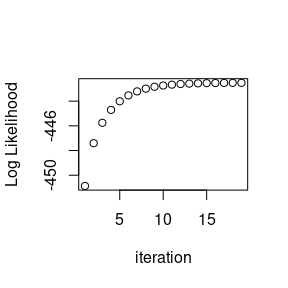Matrix Variate Mixture Models with the t distribution

2019-07-25

library(MixMatrix)

Matrix Variate Mixture Modeling with the $$t$$ Distribution

The matrix variate t distribution was introduced in a previous vignette along with an EM algorithm for maximum likelihood fitting of the parameters. This can be extended rather easily to the case of mixture models for model-based clustering.

As in the case of mixture modeling in general (Fraley and Raftery 2002; McLachlan, Lee, and Rathnayake 2019), the difference in the EM algorithm is that one is now including estimates of $$\pi_{j}$$ for $$j$$ in $$1,2, \ldots, g$$, the estimated probabilities of group membership for the $$g$$ groups in each step and weights $$\tau_{ij}$$, weights for each observation $$i$$ and group $$j$$, where $\pi_j = \frac{1}{n}\sum_{i = 1}^n \tau_{ij}$ and $\tau_{ij} = \frac{\pi_j f(x_i, \Theta_j)}{\sum_{l=1}^g \pi_l f(x_i, \Theta_l)}$

The case of the matrix variate normal distribution can be seen in Viroli (2011), while the case of the multivariate t can be seen in Andrews, McNicholas, and Subedi (2011).

The updates on the parameters $$\Theta$$ are weighted by $$\tau_{ij}$$ in an Expectation/Conditional Maximization algorithm.

Usage

The matrixmixture() function fits unrestricted covariance matrices currently, but future features will implement a teigen type of covariance restriction capability for use with the $$t$$ distribution. It can set means to be constant along rows or columns or both using the row.mean = TRUE and col.mean = TRUE settings.

Currently, this can perform model fitting with unrestricted covariance matrices and fixed degrees of freedom (nu) parameter or for the matrix normal distribution. It does not solve the identifiability problem, that is, that permutations of the labels will yield identical solutions.

matrixmixture function

The function takes data array x, either an argument K for how many groups there are or an initialization of a vector of probabilities prior, an optional initialization of centers and covariance matrices init (if the covariances are left blank, they will be initialized to identity matrices), and optional arguments controlling the other parameters of function, such as number of iterations and normal vs t. If model = "t" is chosen, the degrees of freedom nu must be provided, but in the future it can be estimated.The default method for determining convergence is based on Aitken acceleration of the log-likelihood. However, it can be set to stop based on changes in the log-likelihood instead.

Initialization function

The packages also provides a helper function init_matrixmixture() to provide the init object for you. At present, it can either use the kmeans() function on the vectorization of the input data to provide starting centers or select random points. The ... arguments are passed to kmeans() (so nstart of other similar arguments can be set). If a partially formed init object is sent to the initializer, it will complete it. However, it will not validate that, for instance, the covariance matrices are valid. Partial supply of initial centers is also supported - that is, if fewer centers than groups are provided, the remainder will be chosen by whatever method selected.

init_matrixmixture(C, prior = c(.5,.5), centermethod = 'kmeans')
#> $centers #> , , 1 #> #> [,1] [,2] [,3] [,4] #> [1,] 0.11412574 -0.1191619 -0.04078157 0.127204662 #> [2,] -0.09798116 0.1763393 -0.02998913 0.001676302 #> [3,] 0.16767860 -0.1738115 -0.20268828 -0.079679047 #> #> , , 2 #> #> [,1] [,2] [,3] [,4] #> [1,] 1.0480976 1.0029779 1.0264273 1.0225414 #> [2,] 0.9755583 1.0876427 1.0131553 1.2830619 #> [3,] 0.9418708 0.9333342 0.8656007 0.9025196 #> #> #>$U
#> , , 1
#>
#>      [,1] [,2] [,3]
#> [1,]    1    0    0
#> [2,]    0    1    0
#> [3,]    0    0    1
#>
#> , , 2
#>
#>      [,1] [,2] [,3]
#> [1,]    1    0    0
#> [2,]    0    1    0
#> [3,]    0    0    1
#>
#>
#> $V #> , , 1 #> #> [,1] [,2] [,3] [,4] #> [1,] 1 0 0 0 #> [2,] 0 1 0 0 #> [3,] 0 0 1 0 #> [4,] 0 0 0 1 #> #> , , 2 #> #> [,1] [,2] [,3] [,4] #> [1,] 1 0 0 0 #> [2,] 0 1 0 0 #> [3,] 0 0 1 0 #> [4,] 0 0 0 1 init_matrixmixture(C, K = 2, centermethod = 'random') #>$centers
#> , , 1
#>
#>          [,1]      [,2]      [,3]      [,4]
#> [1,] 1.678144 1.1288934 1.0701532 0.8945011
#> [2,] 1.452156 1.0876125 0.6176221 1.0579284
#> [3,] 1.271707 0.8391508 1.0755241 1.3799744
#>
#> , , 2
#>
#>             [,1]        [,2]       [,3]        [,4]
#> [1,] -0.13670235 -0.22542286 -0.1013062  0.08921306
#> [2,]  0.02554019  0.07765350 -0.1971374 -0.09639281
#> [3,] -0.47626926 -0.03061907  0.1719943 -0.17784713
#>
#>
#> $U #> , , 1 #> #> [,1] [,2] [,3] #> [1,] 1 0 0 #> [2,] 0 1 0 #> [3,] 0 0 1 #> #> , , 2 #> #> [,1] [,2] [,3] #> [1,] 1 0 0 #> [2,] 0 1 0 #> [3,] 0 0 1 #> #> #>$V
#> , , 1
#>
#>      [,1] [,2] [,3] [,4]
#> [1,]    1    0    0    0
#> [2,]    0    1    0    0
#> [3,]    0    0    1    0
#> [4,]    0    0    0    1
#>
#> , , 2
#>
#>      [,1] [,2] [,3] [,4]
#> [1,]    1    0    0    0
#> [2,]    0    1    0    0
#> [3,]    0    0    1    0
#> [4,]    0    0    0    1